# Solving for the Positive Steady State when it Exists¶

$\theta = \sum P(d) \frac{\lambda \theta d^2}{[\lambda \theta d + 1)E[d]]}$

$1 = \sum P(d) \frac{\lambda d^2}{[\lambda \theta d + 1)E[d]]}$

(1) Regular: so just plugging in that everybody has the same degree which is just the expected degree, then we can:

• get rid of $\sum P(d)$
• $d^2 \rightarrow E(d)^2$
• $\lambda \theta d \rightarrow \lambda \theta E(d)$

Regular: $1 = \frac{\lambda E[d]}{(\lambda \theta E[d] + 1)}$

then we can rearrange this in terms of $\theta$

$\theta = 1 - \frac{1}{\lambda E[d]}$

$\theta$ is increasing in $\lambda E[d]$ Need $\lambda E[d] > 1$

So if everybody had the same degree then we can solve explicitly for what the steady state expression is going to be for the infection rate.

$\theta = \sum P(d) \frac{\lambda \theta d^2}{[\lambda \theta d + 1)E[d]]}$

$1 = \sum P(d) \frac{\lambda d^2}{[\lambda \theta d + 1)E[d]]}$

(2) Power law degree distribution: $P(d) = 2d^{-3}$

If plug in the power law P(d) and integrate this out, then end up with an expression that can solve for $\theta$:

$\theta = \frac{1}{\lambda(e^{\frac{1}{\lambda}} - 1)}$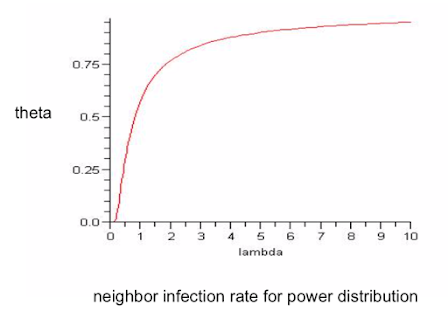As $\lambda$ increases we get a very rapid increase and then eventually it asymptotes, it can't go above "1", but we're getting a very high neighbor infection rate as $\lambda$ increases, because then we've got these very high degree nodes, they become infected, they infect others and so forth, and as $\lambda$ is increasing, we get a very rapid infection increase.

# Ordering Networks - Jackson Rogers (07b)¶

$\theta = \sum P(d) \frac{\lambda \theta d^2}{[\lambda \theta d + 1)E[d]]}$

How does the right side shift with the degree distribution, P(d), if we want to do comparisons, if we go from regular to power law or a regular to Erdos Renyi, sort of graph?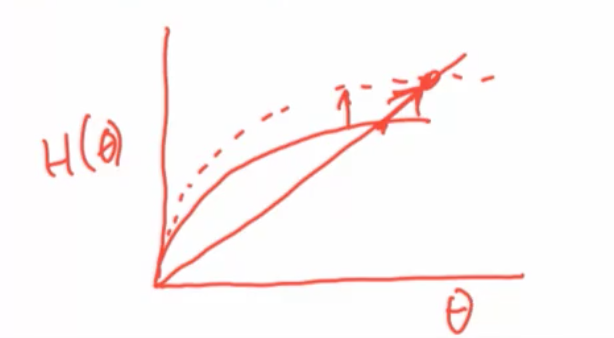Remember the way that we're solving this, we look at theta here, we have this right hand side, which is H(θ) and we're looking for the solution to this thing and if we can say that H(θ) goes up, right, so if we do something that changes H(θ) in a way that goes up then that's going to move the solution to this equation upwards. So, any kind of comparative static where we're making changes that change the distribution in a way that increases this overall expression on the right hand side for each theta gets a higher value then we can say something about what the resulting change is in theta. So, let's see what we can say about how this right hand side moves.

$\frac{\lambda \theta d^2}{[\lambda \theta d + 1)E[d]]}$ is increasing in d

So higher degree nodes are going to have higher relative expected infection rates and basically that's what we're getting

If $P'$ first order stochastic dominates $P$, then rhs increases at every $\theta$

If $P'$ is a mean-preserving spread of P, then rhs increases at every $\theta$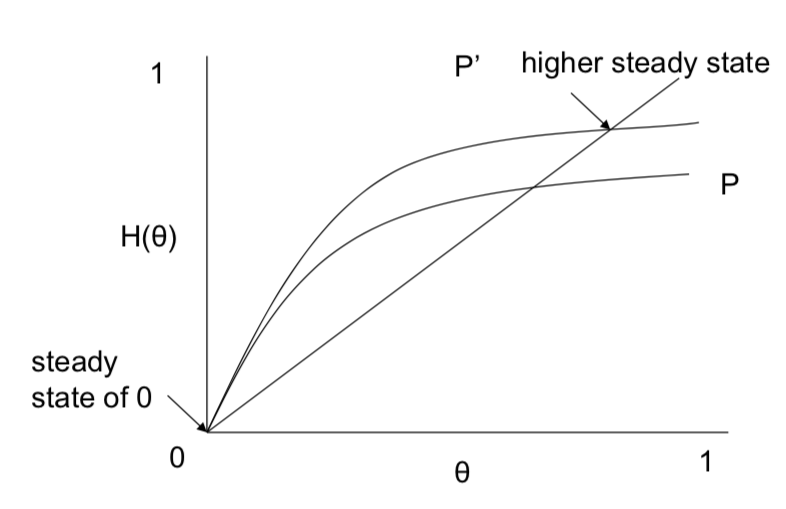# Ideas¶

• Mean preserving spread - more high degree nodes and low degree nodes

• Higher degree nodes are more prone to infection

• Neighbors are more likely to be high degree

• So, either first order stochastic dominance, or mean-preserving spreads in $P$ increase $\theta$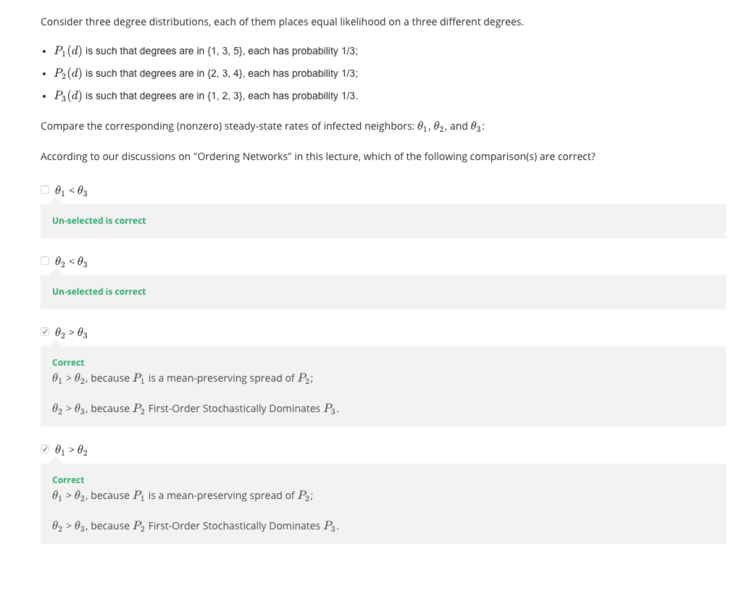# What about Average?¶

• infection rate of neighbors is not the same as infection rate of the population

• $\theta$ is the chance when you're meeting somebody in the population that they're infected, and is increased as we increased in the senses of first or second order stochastic dominance.
• $\rho$ in the population is the acutally average, if we take expectations over all degrees, so the higher people are going to be infected at higher rates, so when you're meeting them at higher values that means that people you're going to meet are more infected, but if we're somebody who just cares about the average level in the population, so if I'm a government and I care about how infected my population is, ultimatly what I care about is what $\rho$ is, not what $\theta$ is, so $\theta$ is very important in determining what the steady state is going to be, but the thing I might be interested in terms of my policies is what fraction of my population ends up being infected
• Theorem JR(2007): If $P'$ is a mean preserving spread of P, then the highest steady state $\theta' > \theta$, but the corresponding $\rho' > \rho$ if $\lambda$ is low, while $\rho' < \rho$ if $\lambda$ is high.

• what's the intuition behind this? The intuition is that in situations where $\lambda$ is very high, the high interaction nodes are already going to be very infected. And so, actually increasing, in putting weight on higher degrees isn't going to matter that much because those nodes are going to be infected at such a high rate that they're going to already be infected. And so you're not changing that much but putting more weight on low degree nodes can actually decrease the, so now you've got some people who have very few interactions. Those people can actually end up being infected with lower rates. So, the actual overall infection rate in the society can be balanced by the fact that you are increasing some of the higher degree nodes but those people are already going to be infected even without this increase and the low degree nodes as you move them towards lower part they can actually end up with lower infection rates and so that counterbalances it. So, when you average across the population, not with the relative frequency of meetings, you actually end up with a decrease in the overall rate.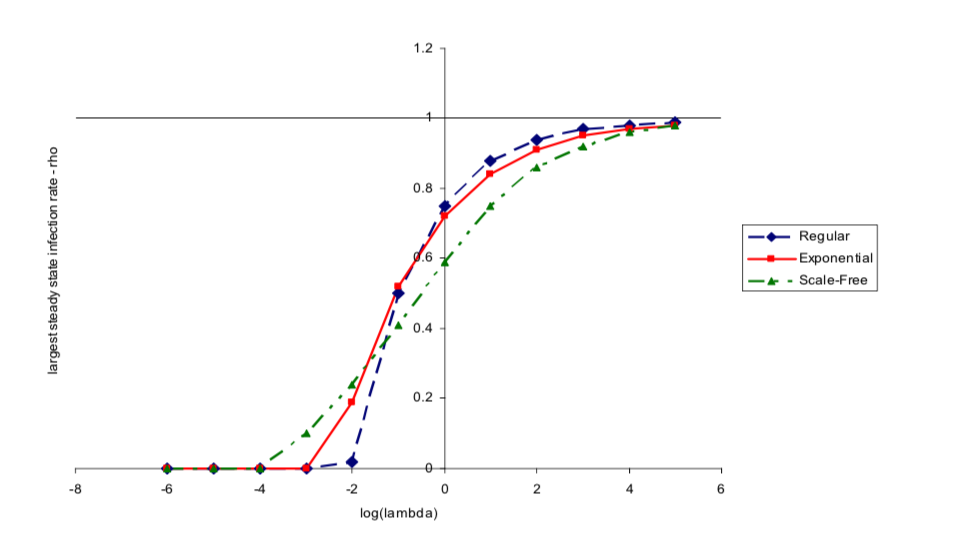# Proof¶

$0 = \frac {d\rho(d)}{dt} = (1- \rho(d))v \theta d - \rho(d)\delta$

Expecting over d:

$0 = v\theta E[d] - \sum P(d)\rho(d) v \theta d - \rho \delta$

$= v\theta E[d] - v \theta^2 E[d] - \rho \delta$

$\rho = \lambda \theta E[d](1-\theta)$

rhs is increasing in $\theta$ iff $\theta < 1/2$, and is decreasing in $\theta$ iff $\theta > 1/2$

$\theta$ is increasing in $\lambda$

# SIS Diffusion Model¶

• Simple and tractable model

• Bring in relative meeting rates

• Can order infections by properties of "network"

The limitationis of this model:

• we lose the fact that a lot of applications are ones where you become infected but then if you recover you're actually immune to catching the disease again, which is actually true of some flus, so it's somewhat limited in term of the applications.

• Also the interactions that we talked about were completely random meeting processes. So, it was not as if we'd drawn out a network and actually had people located on the network. We just had people bumping into each other and meeting each other and so that's a special kind of process which gives rise to special kinds of conditions.

# Next¶

Now we, more generally what we'd have to do if we start to work with things where the network architecture is given, then it's going to be more important to use simulations and so forth. So, we did some calculations before where we talked about component science and so forth and that gives us some insights. But, more generally if we actually want to study these processes a lot of it's going to be done by simulation. So, if you give me a particular network and ask what's going to happen on it then I might have to write down a program and actually simulate what's going to happen there. So, the next thing we'll look at is a simple model of diffusion where we'll do some calculations and just simulate that model and see exactly how it works and that will allow us to actually fit something directly to data. And there's a large amount of that that goes on in epidemiology and marketing and other kinds of areas where you're trying to make predictions. If you know something about the network you're working with you can actually simulate things and that's going to go a long way towards improving your accuracy. So, the SIS model gives us nicer intuitions, simple ideas, but it's not one you're going to easily take to data. We're going to have to enrich the model to fit it to networks. And that's what we'll talk about next.